## 1 Introduction

Programming languages and compilers help engineers describe each system at the most expedient level of abstraction. The process of experimenting with new languages is most familiar from the software world, but hardware designers also benefit from it. Of course, Verilog and VHDL themselves are significant steps up from direct circuit descriptions. Some families of hardware languages go further, in roughly the sense that, say, Java goes further than C, providing abstractions that simplify reasoning about modular design. The rule-based hardware languages like Bluespec  allow hardware designers to imagine that system modules take turns executing local atomic state-change rules, with no concurrency. In reality, parallel execution is essential for performance, and compilers for these languages rely on static analysis to extract parallelism soundly.

Roughly speaking, a rule in Bluespec and its relatives must run within a single clock cycle. What happens when we want to simplify reasoning about longer-running processes? A prime example is a cache-coherence protocol. A memory hierarchy is a distributed system, with many caches communicating through explicit message passing, requiring at least as many clock cycles as the longest dependency chain of message exchanges. The logic is notoriously difficult to get right. One reason is that many memory requests from processor cores may be handled simultaneously. One cache may be working on one request, while a neighboring cache is working on a different request. Might there be abstractions that remove this complication from the hardware designer’s thought process, much as Bluespec allows the same designer to pretend that different hardware components do not execute state-change logic in parallel?

We answer affirmatively in presenting Hemiola, the first hardware-description language that presents cache-coherence transactions as if they run atomically, while realizing the usual parallel performance gains. We define a transaction as all the activity within the memory system in response to a single request from a processor core or other user of the memory. One request may trigger a flurry of activity in the protocol, but the designer may at least pretend that no other request is active in the same period.

The foundation of Hemiola is identifying commonalities across practical cache-coherence protocols and embodying them in a domain-specific language (DSL). We fix a notion of node hierarchy and message-passing channels, enumerating rule templates capturing relevant communication patterns. Protocols are then described in terms of single-cycle, per-cache rules, each instantiated from a template. Crucially, a locking discipline is built into the language and handled automatically by the templates.

In addition to the DSL, Hemiola provides formal tools significantly easing verification of all cache-coherence protocols designed in it. The DSL is embedded in the Coq proof assistant and has a fully machine-checked proof of soundness, formalized as serializability: any state invariant preserved with one-transaction-at-a-time execution is also preserved in true parallel execution. The serializability property is once-and-for-all at the language level, freeing protocol designers from needing to reason about interleavings among transactions. In a sense, our work takes techniques that have been used for per-protocol verification and lifts them to apply at the level of a DSL, so that no verification effort need be expended on them per-protocol.

To sum up, the contribution of this paper consists of two partsFootnote 1:

• We discover a set of topology and lock conditions that ensures serializability, extracted from usual cache-coherence protocol designs. We then identify a DSL, where every protocol defined in this language ensures serializability by-construction, backed up with mechanized Coq proof (Sect. 3). Lastly, we formalize how serializability helps prove global invariants, by using the novel notion of predicate messages in distributed protocols (Sect. 4).

• We provide the complete correctness proofs of hierarchical cache-coherence protocols (Sect. 5) using Hemiola. Our case studies are the first complete mechanized proofs that share a large segment of reusable proofs across various cache-coherence protocols. We also demonstrate that the case-study protocols are hardware-synthesizable, by using a compilation/synthesis toolchain in Hemiola (Sect. 6).

## 2 A Motivating Example

Before introducing our proposed method to design and verify cache-coherence protocols, we provide a simple motivating example to explain the typical challenges and how we suggest to handle them. For simplicity, in this section, we will consider a protocol handling only a single memory location. We will see it is still nontrivial to design a correct protocol.

The overall goal of cache coherence is to preserve coherence among multiple candidate values in a memory subsystem. In other words, if the system is coherent, then it should behave like an atomic memory. Figure 1 shows caches and network channels for a directory-based MSI protocol. There are three caches (P, $$C_1$$, and $$C_2$$), and each of them has its own status (Modified, Shared, or Invalid) and data (v). In this MSI protocol, a cache can read/write the data with the M status, only read with S, and cannot read/write with I. The parent P additionally has a data structure called a directory to track the statuses of the children. For example, a directory might be $$S_{\langle 1, 2 \rangle }$$, meaning that both $$C_1$$ and $$C_2$$ have S status, in some logical snapshot of state.

Caches communicate through ordered channels, shown as $$(\rightarrowtail )$$ in the figure. Child caches ($$C_1$$ and $$C_2$$) have channels to receive and respond to requests from processor cores. There are three types of channels between a parent and a child: one channel is for parent-to-child messages, and the other two channels are for child-to-parent requests and responses. It is natural to wonder why two separate child-to-parent channels are required; we will see the reason very soon.

Figure 1 also depicts some example state-transition cases depending on the cache statuses. In this setting, all the caches run concurrently by repeatedly executing rules that make atomic, local state transitions. A rule may take some messages from input channels, perform a state transition, and put messages in output channels. A rule may also have a precondition, blocking use of that rule when the precondition does not hold.

A rule execution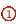is a case where a child $$C_1$$ takes a request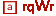from a processor to write data, but it does not have M status and thus further requests to the parent () to get the permission. At this moment, in many practical cache-coherence-protocol designs, $$C_1$$ changes its status to a transient state SM to record its current status (S) and the next expected status (M) and to make any further processor requests stall.

Due to the concurrent execution of caches, we might have another rule executed at the same time.is executed concurrently with, where $$C_2$$ also takes a processor request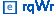and sendsto the parent as well. Sinceand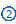happened at the same time, the parent P needs to decide which request to deal with. Suppose that it decided to handlefirst.presents the next execution by P, taking the input message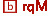and making an invalidation request () to the other child $$C_2$$ to change its status to I. This request is required, since when a child has M, the others should not be able to read/write the data. The parent, at this moment, changes its directory status to a transient state to disallow any other requests from the children (e.g.,), since otherwise it will handle two $$\textsf {rqM}$$ messages simultaneously, which might lead to an incoherent state – two M statuses in the caches.

Lastly,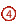shows the case that $$C_2$$ handles the invalidation request (). A number of corner cases should be handled carefully in this step:

• Since $$C_2$$ requested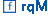, it has a transient state SM whenarrives. It should still be able to handle this invalidation request even in the transient state (while any processor requests stall). In this case, $$C_2$$ accepts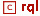and changes its transient state to IM. We see that transient states should be fine-grained enough to distinguish which requests to handle.

• Due to the existence of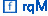, if we had a single channel from a child to a parent, a deadlock would occur. P cannot takesince it is in a transient state after making an invalidation request. It cannot takeas well, since the response is not at the head of the ordered channel. This case shows the necessity of having multiple channels between a child and a parent.

A so-called three-channel system has been widely used and regarded as a good choice to make the design correct and live [33, 34]. While there are other possible correct topology and network settings, the cases shown in Fig. 1 at least demonstrate that it is nontrivial to construct one of them. Note that the three-channel system is logical in the sense that the actual hardware implementation may use various hardware components that can simulate the requirements.

In terms of making a protocol design correct, transient states, topology, and network settings contribute to make interleavings correct. Considering the sequence of rule executions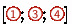(in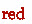) as an execution flow – we will later call it a transaction – to handle a processor request, we see that the other execution flow (in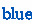) could not happen after, which is for another processor request. As explained above case-by-case, proper transient states and network channels madestall. This mechanism to ensure safe interleavings is called noninterference [11, 18], which ensures that no other transactions spuriously affect state transitions by an ongoing transaction.

Hemiola in a Nutshell. If transient states, proper topology, and network settings are essential for designing a correct protocol, can we craft a DSL where only conformant protocols are expressible?

That is exactly what we did with Hemiola. The Hemiola DSL helps designers design cache-coherence protocols in a safe way. Instead of requiring designers to use transient states coupled to a protocol, we discover general stall conditions that by themselves ensure noninterference and form those conditions as conceptual locks. The stall conditions are extracted and abstracted from the usual transient states, so they can apply to practical protocols.

For instance, a designer may write a rule forwithout any DSL support like the left rule in the following code:

Note that a designer has to find proper input/output channels (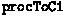and) and check/set a proper transient state () in order to define the rule.

On the other hand, the left rule can be written more easily by using the Hemiola DSL as the right rule. Instead of using explicit channels and transient states, the right rule just uses the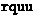rule template (wherestands for request-up-up). The rule templates employ proven-safe network structures and automatically check/set/release associated locks, so users can design protocols without worrying about incorrect use of network channels, locks, etc.

## 3 The Hemiola Domain-Specific Language

As explained in Sect. 2, in designing a cache-coherence protocol, it is nontrivial to make concurrent execution of transactions correct. In this section, we introduce the Hemiola DSL to ease that burden. While conventional approaches deal with transient states directly to derive noninterference per-state, the Hemiola DSL limits protocols to satisfy abstract conditions that can guarantee noninterference by-construction. The conditions have already been mentioned in Sect. 2 – network topology and locking mechanisms extracted from transient states of practical cache-coherence protocols.

Notations. An overline (e.g., $$\overline{l}$$) denotes a list. $$[]{}$$ and $$(\overline{l} + e)$$ denote nil and single-element append, respectively. $$\oplus \overline{l}$$ flattens the list of lists $$\overline{l}$$ with repeated concatenation. $$(\overline{l_1} + \overline{l_2})$$, $$(\overline{l_1} - \overline{l_2})$$, and $$(\overline{l_1} \; \# \; \overline{l_2})$$ denote append, subtraction, and disjointness of lists, respectively. We use the same operation $$(+)$$ for the single-element and general append. Regarding a list of key-value pairs as a finite map, we override notations for lists. For example, $$(M + \overline{l})$$ updates multiple key-value pairs in a finite map M. Moreover, we overload the same operation $$(M + (k, v))$$ for a single update for simplicity. $$(\overline{s.\textsf {fd}})$$ is used as a shorter notation for $$(\textsf {List.map}\ (\lambda s.\; s.\textsf {fd})\ \overline{s})$$. We use $$\langle \cdot \rangle$$ to denote a struct and use a name (e.g., $$s.\textsf {fd}$$) to access a field value.

### 3.1 Syntax

The Hemiola DSL is similar to well-known rule-based hardware-description languages (HDLs) such as Bluespec , Kami , and Kôika . A notable difference is that rule descriptions are restricted by predesigned rule templates to avoid spurious interleavings among transactions.

A system $$S\,{:}{:}{=}\, \langle \overline{C}, \overline{i_{\text {in}}}, \overline{i_{\text {rq}}}, \overline{i_{\text {rs}}} \rangle$$ is the biggest unit of the language; it consists of caches ($$\overline{C}$$) and channel indices for internal messages ($$\overline{i_{\text {in}}}$$) and external (processor) inputs($$\overline{i_{\text {rq}}}$$)/outputs($$\overline{i_{\text {rs}}}$$). A cache $$C\,{:}{:}{=}\, \langle i, s_{\textsf {init}}, \overline{r} \rangle$$ consists of its index (unique within a system), an initial state ($$s_{\textsf {init}}$$), and rules ($$\overline{r}$$). A rule (r) makes state transitions within the cache, and it is always defined by one of the rule templates provided by the language.

Each rule template must be instantiated with a rule index (should be unique within a cache), a precondition, and a transition function, where the types of the precondition and transition vary by template. A precondition of a rule template usually takes input messages and a (partial) current cache state and decides whether the rule can be executed or not. A transition function takes the same arguments in general but returns the next cache state and output messages. Neither state transition nor input-messages consumption happens if the precondition does not hold. We will introduce the detailed rule-template forms in the next section (Sect. 3.2).

A message $$m\,{:}{:}{=}\, \langle \textsf {ty}, \textsf {id}, \textsf {val} \rangle$$ is composed of a Boolean message type (request or response), a message ID (effectively from an enumeration of message kinds), and a value. We use value to refer to the set of legal contents of memory addresses. A pair $$im\,{:}{:}{=}\, (i, m)$$ is used sometimes to represent a message m in a channel with an index i.

### 3.2 Rule Templates

The Hemiola DSL follows syntax and semantics of traditional rule-based HDLs, but the major difference is that Hemiola further restricts the way of describing rules, which itself guarantees noninterference among transactions.

Topology and Network Requirements. First of all, Hemiola requires that the caches in a given system form a tree topology. Most cache-coherent memory subsystems follow this topology, where leaf nodes correspond to L1 caches, and the root corresponds to the main memory. A child and its parent in the tree communicate using the three channels shown in Sect. 2.

Note that the topology and network settings are required logically; the actual hardware implementation may use various hardware components (e.g., finite-capacity FIFOs or buses) that can simulate the requirements.

Locking Mechanism. We saw in Sect. 2 why transient states are required to ensure noninterference in cache-coherence protocols. Revisiting the issue described in Fig. 1, a child should be able to handle an invalidation request from the parent even if it is in a transient state (SM), and after handling the request it changes its transient state to IM.

Hemiola supports a locking mechanism reflecting this discovery; the locking is more general in that the framework looks at whether the message is from the parent or a child. This mechanism is still enough to describe practical cache-coherence protocols and sufficient to ensure noninterference.

In particular, Hemiola employs two kinds of locks: uplocks and downlocks. We say a cache is uplocked (or downlocked) when it holds an uplock (or downlock), respectively. Figure 2 depicts the locking mechanism in Hemiola. An uplock is set when a cache ($$P_1$$ in the figure) makes an upward request to its parent ($$P_2$$); it is released when the cache gets a corresponding response from the parent. The cache cannot make any further upward requests while uplocked. On the contrary, a downlock is set when a cache ($$P_2$$ in the figure) makes a downward request(s) to some of its children; similarly it is released when the cache gets corresponding response(s) from the child requestee(s). The cache cannot make any further downward requests while downlocked.

Now every cache defined in Hemiola does not need to set transient states to consider all possible combinations among stable statuses. For instance, instead of setting a transient state SM, it is now desirable to maintain its status S and set an uplock to record it just made an upward request. We emphasize that the Hemiola locks do not enforce more restrictions on protocols than what is enforced by transient states; e.g., as an uplock makes certain messages like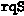and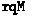stall, a transient state SM makes them stall as well.

Each cache defined by Hemiola has a semantic lock state holding a lock type (uplock or downlock) and related messages/indices. The user, however, does not need to deal with this lock state while using the DSL; locks are managed implicitly by Hemiola.

Note that the DSL supports design of single-cache-line protocols, and thus the uplock and downlock are assigned per-line. The single-line protocol is then naturally extended to all cache lines using a protocol compiler that will be introduced in Sect. 6. This approach is sound in terms of correctness, since a transaction does not affect coherence for the other lines.

The Nine Rule Templates. Hemiola provides a set of rule templates for describing protocols in a way that guarantees noninterference by-construction. Figure 3 presents the nine rule templates. Each diagram has the form $$\{P\}C[Q]$$ and arrows (representing the directions of messages; e.g., a downward arrow indicates messages from a parent) with circles ($$\circ$$ for inputs and $$\bullet$$ for outputs) and labels representing requests ($$\textsf {rq(s)}$$) and responses ($$\textsf {rs(s)}$$). It means that the rule template is for a cache C, requires input messages ($$\circ$$) with the message types determined by the label, has a precondition P, performs a state transition Q, and generates output messages ($$\bullet$$). The precondition and state transition are implicit in the sense that they are automatically checked and performed, respectively, whenever the rule is executed. Note that some rule templates may make local state transitions without any input/output messages (input/output messages marked with parentheses in Fig. 3).

$$\textsf {UL}$$, $$\textsf {DL}$$, $$!\textsf {UL}$$, and $$!\textsf {DL}$$ in a precondition indicate that the cache should be uplocked, downlocked, uplock-free, and downlock-free, respectively. $$\textsf {UL}\!\Uparrow$$, $$\textsf {DL}\!\Uparrow$$, $$\textsf {UL}\!\Downarrow$$, and $$\textsf {DL}\!\Downarrow$$ in a state transition indicate setting an uplock, setting a downlock, releasing an uplock, and releasing a downlock, respectively. $$\textsf {SLT}{}$$ annotates that the rule template forbids any state modification beside locking.

The rule templates are carefully designed to avoid any spurious interleavings among transactions. We see a number of cases that are worth analyzing:

• immu and rqdd show that a cache can handle a downward request even when uplocked. These rules do not have a precondition that the cache should be uplock-free. This relaxation is necessary to avoid a deadlock.

• rsdd says that in order to handle a response from the parent, the cache should be downlock-free. This precondition is required to ensure noninterference.

• rsrq forces the order of a traversal, saying that the traversal for the outer caches must be done before traversing the inner caches. This rule is used when a transaction needs to traverse all the caches in the system, e.g., invalidating all the other caches to obtain the M status. The forced order is important to avoid a deadlock.

## 4 Verification in Hemiola

We have introduced the Hemiola DSL in Sect. 3 and provided an intuition that rule templates ensure general noninterference, i.e., interleavings among any transactions are safe. That said, we have not yet showed how the rule templates guarantee such noninterference in a formal way. We also have not explained how noninterference eases the verification of cache-coherence protocols.

In this section, we provide the semantics of the Hemiola DSL and the formal meaning of general noninterference called serializability. We then introduce our novel approach to proving invariants called predicate messages, which eliminates the burden of considering interference while proving invariants.

### 4.1 Semantics of the Hemiola DSL

A system in Hemiola follows so-called “one-rule-at-a-time semantics” [4, 5, 10, 34], i.e., any state transition by concurrent rule executions can be interpreted as a serial execution of rules. Thus, it is fair to consider that a state transition happens by executing a single rule.

Transition Steps. Figure 4 describes the complete semantics for transition steps of the Hemiola DSL. The semantics for a step is presented as a judgment $$s_0 \mathop {\rightarrow }\limits _{S}^{l} s_1$$, where S is the system to execute, $$s_0$$ is a prestate, $$s_1$$ is a poststate, and l is a label generated by the state transition. The state of a system (in domain $$\mathbb {S}{}$$) is a pair $$\langle \overline{c}, M \rangle$$ of cache states ($$\overline{c}$$) and message states (M). Cache states are represented in a finite map from cache indices to cache states, and message states are represented in a finite map from channel indices to ordered queues of messages.

Rule [SSilent] represents the case where no state transition happens in the current step; an empty label ($$l_\epsilon$$) is generated in this case. From now on, we assume that all the input/output messages used in the step definitions do not share the same channel, i.e., $$(\textsf {List.NoDup}\, \overline{im.i})$$. [SIns] describes the case for external input messages coming to the system; an external-inputs label ($$l_{\text {in}}(\overline{im})$$) is generated in this case. [SOuts] describes the opposite case, for output messages being released to the external world, generating an external-outputs label ($$l_{\text {out}}(\overline{im})$$).

Lastly, [SInt] deals with a state transition by a rule (r) in a cache (C). It nondeterministically chooses a cache and a rule in the cache, checks that the precondition holds, and applies the transition to update the state of the system; an internal label ($$l_{\text {int}}(C.i, r.i, \overline{im^{\text {ins}}}, \overline{im^{\text {outs}}})$$) is generated in this case, which records a cache index, a rule index, input messages, and output messages. Note that the semantics is based on ordered channels, so messages are enqueued and dequeued in each state-transition case.

The step semantics is naturally lifted to one for multiple steps, presented as a judgment $$s_0 \mathop {\Rightarrow }\limits _{S}^{\overline{l}} s_1$$, where $$\overline{l}$$ is a sequence of labels generated by executions of the steps in order. We will sometimes call such a sequence of labels a history.

We say that a state s is reachable iff there is a history $$\overline{l}$$ such that $$S_{\textsf {init}} \mathop {\Rightarrow }\limits _{S}^{\overline{l}} s$$ holds, where $$S_{\textsf {init}}$$ is the initial state of the system S. We use a simpler notation $$S \Rightarrow s$$ for reachable states. We also call such a history $$\overline{l}$$ legal, denoted as $$S \mathop {\Rightarrow }\limits ^{\overline{l}} \bullet$$. We call $$\mathcal {I}: \mathbb {S}{} \rightarrow \mathbb {P}{}$$Footnote 2 an invariant over a system S if $$\mathcal {I}$$ holds for all reachable states, i.e., $$\forall s.\; (S \Rightarrow s) \rightarrow \mathcal {I}(s)$$.

Behaviors and Correctness. A system S has a behavior $$\lfloor \overline{l} \rfloor$$ (denoted as $$S \Downarrow \lfloor \overline{l} \rfloor$$) iff $$S_{\textsf {init}} \mathop {\Rightarrow }\limits _{S}^{\overline{l}} s$$ holds, where $$\lfloor \cdot \rfloor$$ filters out silent ($$l_\epsilon$$) and internal ($$l_{\text {int}}$$) labels so only the external parts remain. We call such a sequence of labels a trace. Lastly, we say that a system I (“implementation”) trace-refines another system S (“specification”), written as $$I \sqsubseteq S$$, iff every trace of I is also a trace of S:

\begin{aligned} I \sqsubseteq S \triangleq \forall \overline{t}.\; I \Downarrow \overline{t} \rightarrow S \Downarrow \overline{t}. \end{aligned}

In order to prove trace refinement, we usually establish a simulation relation  between the implementation and the spec states and prove that the relation is preserved over steps, and it is crucial to state and prove proper invariants of the implementation for the simulation proof. Since the invariant proof is indeed the most significant part of the whole correctness proof, in this paper we would like to focus on how Hemiola helps a user state and prove invariants.

### 4.2 Serializability in Hemiola

Serializability [3, 28] is a celebrated notion of concurrency correctness. While each transaction in a system affects multiple values, serializability guarantees that interleaved execution of such transactions is correct in that the effect (state change) is the same as if the transactions were executed serially, i.e., atomically in some order with no interleaving.

In order to define serializability formally, we first provide basic definitions of atomic histories and transactions. A history h is atomic iff it satisfies the predicate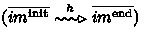with initial messages $$\overline{im^{\text {init}}}$$ and live messages $$\overline{im^{\text {end}}}$$, constructed inductively by the following two cases:

• Any singleton history with an internal label is an atomic history with its input and output messages as initial and live messages, respectively.

• If h is an atomic history, $$(h + l)$$ is also an atomic history if l consumes its input messages from the live messages of h. The new live messages are constructed by subtracting the input messages and adding the output messages of l to the previous live messages.

Figure 5 presents an atomic history already shown in Fig. 1. h is generated by executions of three rules, $$r_1 \in C_1.\overline{r}$$, $$r_2 \in P.\overline{r}$$, and $$r_3 \in C_2.\overline{r}$$. Rule $$r_1$$ takes an input message $$(1, \textsf {rqWr})$$ (from the channel with index 1) as an initial message of the history. Rule $$r_2$$ takes $$(3, \textsf {rqM})$$, the output message from $$r_1$$. Finally, $$r_3$$ takes $$(8, \textsf {rqI})$$, the output message from $$r_2$$. Summing up all the rule executions, by the definition of an atomic history we get the predicate lower-right in Fig. 5.

This example shows that an atomic history intuitively captures a transaction flow triggered by the initial messages. Note that an atomic history does not need to be completed, e.g., h in the example is incomplete in the sense that the live message ($$\textsf {rsI}$$) is not a response sent to an external channel.

We call an atomic history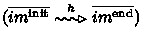a transaction if its initial messages are external requests ($$\overline{im^{\text {init}}.i} \subseteq S.\overline{i_{\text {rq}}}$$); we denote it as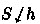.

With a clear notion of transactions, we can now easily define sequential histories and serializability. A history h is sequential iff the history is a concatenation of transactions: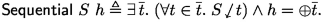A legal history h is serializable in the system S iff there exists a sequential history that reaches the same state:

\begin{aligned} \mathsf {Serializable}\ S\ h \triangleq \; \forall s.\; S_{\textsf {init}} \mathop {\Rightarrow }\limits _{S}^{h} s \rightarrow \exists \, h_{\text {seq}}.\; \mathsf {Sequential}\ S\ h_{\text {seq}} \wedge S_{\textsf {init}} \mathop {\Rightarrow }\limits _{S}^{h_{\text {seq}}} s. \end{aligned}

A system S is serializable iff every legal history is serializable:

\begin{aligned} \mathsf {Serializable}\ S \triangleq \forall h.\; \mathsf {Serializable}\ S\ h. \end{aligned}

### 4.3 Predicate Messages

Now we discuss how to exploit our notion of serializability: how does it help prove global invariants of a system? In proving the correctness of a cache-coherence protocol, it is very common to state an invariant like “an important property holds whenever the system includes a certain message in a certain channel.” We call such an invariant a predicate message, giving the intuition of messages that logically carry predicates that must be true so long as those messages remain in play. More formally, $$S \vdash im\{P\} \triangleq \forall s.\; (S \Rightarrow s) \rightarrow im \in s.M \rightarrow P(s)$$, where s.M refers to the message state of the system. We will write just $$im\{P\}$$ when the system S is clear from context, also often using a shorter version $$\textsf {id}{}\{P\}$$ (considering only messages with a given ID) when it is not ambiguous.

Figure 6 presents an example of a predicate message. When a child $$C_2$$ is about to handle a response message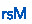, which is a permission to change the cache status to M, we expect the parent and the other child $$C_1$$ to have I status (likein the figure). However, between the sending of that message and receipt by $$C_2$$, the predicate may be broken by another transaction; for instance, the predicate no longer holds if a state transition happens by, which takes anotherand updates the status of $$C_1$$ to M.

Investigating this corner case carefully, we find that actually no two different $$\textsf {rsM}$$ messages can be in the system at the same time. It implies that now the predicate message for $$\textsf {rsM}$$ should have a much-more-complicated form, which considers all possible noninterference cases. The complete desired predicate message for $$(8, \textsf {rsM})$$ will then look like:It is indeed a burden to consider all possible interleavings per predicate message. We would not have faced such a complication if we could ensure that no other transactions interfere while handling a transaction. Serializability guarantees exactly that simplification, and Hemiola provides a way of designing and proving predicate messages in the simpler form, not taking any interference into account.

Our novel approach to employing predicate messages in atomic histories begins with formalizing the notion of atomic invariants. We say that $$\mathcal {I}_A: \overline{\mathbb {I}\mathbb {M}{}} \times \mathbb {S}{} \rightarrow \mathbb {P}{}$$ is an atomic invariant iff $$\mathcal {I}_A\, (\overline{im_o},\, s_1)$$ holds for any atomic history h with $$s_0 \mathop {\Rightarrow }\limits _{S}^{h} s_1$$ and.

Figure 7 shows an example of predicate messages defined in an atomic history, formalized as an atomic invariant. An atomic invariant $$\mathcal {I}_A$$ is a conjunction of clauses $$(im \in \overline{im_o} \rightarrow P(s))$$, each claiming that the predicate P holds when im is in the live messages $$\overline{im_o}$$. We can prove that the atomic invariant $$\mathcal {I}_A$$ holds by induction on state-transition steps through the atomic history in the figure:

• The initial step of the atomic history is the one by $$r_1$$. The live messages are $$[(4, \textsf {rsI}) ]$$. Since $$r_1$$ changes the status of $$C_1$$ to I, it is straightforward to prove $$\mathcal {I}_A$$.

• The next step is by $$r_p$$, and at this point the live messages are $$[(8, \textsf {rsM}) ]$$. By the induction hypothesis, we obtain the predicate message $$(4, \textsf {rsI})\{C_1.\text {st} = I\}$$. Since $$r_p$$ changes the status of P to I, we can prove the predicate for $$(8, \textsf {rsM})$$.

• The last step is by $$r_2$$, and the live messages are $$[(10, \textsf {rsWr}) ]$$. $$\mathcal {I}_A$$ trivially holds here since it does not contain any predicate for $$(10, \textsf {rsWr})$$.

Note that the invariant proof was straightforward since no other state transitions interfere with an atomic history.

How do atomic invariants help prove conventional invariants? If the system S is serializable, by definition, for every reachable state there is a sequential history that reaches the same state. Since the sequential history is a concatenation of transactions, an invariant can be proven by showing that any transaction preserves it.

Since a transaction is an (external) atomic history, we can make use of corresponding atomic invariants. In other words, we can employ both conventional/atomic invariants ($$\mathcal {I}$$ and $$\mathcal {I}_A$$) to prove the ones for the next state ($$s_{i+1}$$):

\begin{aligned} \mathcal {I}_A\, (\overline{m_i}, s_i) \wedge \mathcal {I}(s_i) \rightarrow (\mathcal {I}_A\, (\overline{m_{i+1}}, s_{i+1}) \wedge \mathcal {I}(s_{i+1})). \end{aligned}

For instance, in proving a cache-coherence protocol, we usually want to have an invariant claiming that at most one node of the system has M status at a time. The predicate messages defined in Fig. 7 will play a crucial role here, e.g., the one for $$(8, \textsf {rsM})$$ says that $$C_1$$ and P both have I status, which means that the state transition by $$(r_2: C_2.\text {st} \leftarrow M)$$ preserves the invariant. We will see more comprehensive uses of predicate messages in our case studies (Sect. 5).

### 4.4 Serializability Guarantee by the Hemiola DSL

The biggest contribution of the Hemiola framework includes the serializability proof. The highest-level theorem simply claims that use of good topology ($$\mathsf {OnTree}\ S\ t$$) and the rule templates ($$\mathsf {GoodRules}\ S\ t$$) guarantees serializability:

\begin{aligned} \forall S, t.\; \mathsf {OnTree}\ S\ t \wedge \mathsf {GoodRules}\ S\ t \rightarrow \mathsf {Serializable}\ S. \end{aligned}

In the proof we used a well-established technique called commuting reductions , showing that any interleaving transactions can be serialized by performing a finite number of reductions. Interested readers are referred to Choi’s dissertation , which describes more details of the proof.

## 5 Case Studies: Hierarchical MSI and MESI Protocols

In this section we explain how we designed, specified, and formally proved the correctness of the following three hierarchical cache-coherence protocols: inclusive/noninclusive MSI protocols and a noninclusive MESI protocol. Each protocol is parameterized by a tree that decides the topology of the memory subsystem. In other words, whenever we instantiate the tree parameter, we get a cache-coherence design and its correctness proof for free.

The protocols are directory-based and support arbitrary evictions. The inclusive MSI protocol requires back-invalidation to maintain the cache-line inclusion . The noninclusive protocols employ the noninclusive-cache inclusive-directory (NCID)  structure to optimize cache space.

We will introduce common points among our case-study protocols. Particularly, we focus on how predicate messages are used (introduced in Sect. 4.3) to ease the invariant proofs required to prove protocol correctness. More details about the correctness proofs are provided in Choi’s dissertation .

### 5.1 Cache States

A cache state consists of a status, a value, a directory, and a Boolean called an ownership bit. A status is either $$\texttt {M}$$, $$\texttt {E}$$, $$\texttt {S}$$, or $$\texttt {I}$$. The MESI protocol applies further optimizations to the MSI protocol: if a cache line has $$\texttt {E}$$ status, then the line is exclusive to the cache but also clean.

A directory contains a status of its children called a directory status and a list of child-cache indices that have the directory status. An L1 cache does not have a directory since it has no children.

The ownership bit decides whether the cache is responsible for writing the value back to the parent when evicted. The ownership bit intuitively constrains which caches can have valid status; we will see how this intuition is formalized as an invariant in Sect. 5.3.

### 5.2 Protocol Description with Rule Templates

We present a number of rule descriptions, used in our case studies, that employ the rule templates provided in Hemiola. Each rule template is defined in Coq, taking in several parameters and generating a rule. We exploited Coq’s notation mechanism to define each rule template compactly.

The above code presents an actual rule definition in an L1 cache, starting with an invocation of a particular rule template, which takes an upward request (to the cache) and sends a further request to the parent. This rules a message with the IDfrom the processor coreFootnote 3 to get a write permission. This rule template also requires to write down the precondition (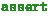) and the output message (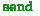). In this example the cache simply forwards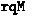to the parent. As explained in Sect. 3.2, therule template does not allow any state transition except locking – the template automatically sets an uplock.

The above rule presents another case that sends the response to the child who requested. Templatesays that the rule takes responses from children and responds back to the original child requestor. The rule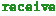s the response message with the ID. In order to execute this rule, the cache should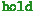a downlock containing the index of the original requestor (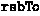) and the request message with the ID, acting like an assertion for the lock state.

As a state transition, this rule sets its status to $$\texttt {I}$$, sets the directory status to $$\texttt {M}$$ by adding the requestor, and sets the ownership bit as false since the requestor will make the value dirty after it obtains $$\texttt {M}$$. It alsos a response () to the requestor. Lastly, the downlock is released automatically and implicitly by therule template.

The above rule is for the MESI protocol, fired when an intermediate cache gets a request from a child to read the data, while the parent has status $$\texttt {E}$$ or $$\texttt {M}$$. In this case, instead of responding with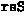, the cache sendsto provide $$\texttt {E}$$. Once the original requestor obtains $$\texttt {E}$$ status, it can both read and write.

### 5.3 Invariant Proof Using Predicate Messages

Now we present how predicate messages (introduced in Sect. 4.3) are used to prove a nontrivial invariant required for all of our three case-study protocols.

Figure 8 shows a coordination between predicate messages and conventional invariants. Suppose that an L1 cache (shown as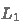in gray in the figure) requested to the parent to get the $$\texttt {M}$$ status. When it finally handles the response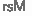, it should know all the other caches (except itself) have been invalidated to prove the desired invariant about $$\texttt {M}$$ (denoted as). This proof case can be supported using the predicate message for $$\textsf {rsM}$$, stating $$\textsf {Invalid}\;(\text {tr}^{-1}\,(C))$$ (the caches outside of the subtree rooted to C are invalid) when the message goes to C. Since $$L_1$$ is a leaf node in the tree, it is trivial to prove $$\textsf {Invalid}\;(\text {tr}^{-1}\,(L_1)) \rightarrow \textsf {Invalid}\;(\lambda c. \; c \ne L_1)$$, so we see an example of a predicate message helping prove a conventional invariant.

Figure 8 also shows another coordination to prove a predicate message. When a child $$C_i$$ sends the invalidation response, it should know that all the caches inside the subtree of $$C_i$$ have been invalidated (denoted as). When the parent P subsequently handles the responses, it responds with $$\textsf {rsM}$$ to the original requestor ($$C_0$$ in the figure), requiring to prove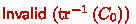, the predicate message for.

While P also changes its status to $$\texttt {I}$$ in this state transition, how do we infer that the caches outside P have already been invalidated, which is required to prove the predicate over? In this case, we should know that 1) P has the ownership bit true (from a simple cache-level invariant of P) and 2) the caches outside of a cache with ownership bit set should have $$\texttt {I}$$ status (denoted as) as an invariant. Combining all the predicates and the state transition by P, we can prove the next predicate message forto the original requestor $$C_0$$.

## 6 Compilation and Synthesis to Hardware

So far we have dealt with cache-coherence protocols for a single line. In order to build a hardware-synthesizable multiline implementation, we developed a compiler that takes a single-line Hemiola protocol and generates a multiline implementation described in Kami .

Kami is a hardware formal-verification framework, where its own HDL and proof tools are defined in Coq, allowing users to design, specify, verify, and synthesize their hardware components. Since Kami already has a hardware-synthesis toolchain, we can just compile a Hemiola program to Kami and use the toolchain to run it on FPGAs.

### 6.1 Compilation of Hemiola Protocols

The compiler uses prebuilt hardware components described in Kami. One of them is NCID , whose interfaces include asynchronous read and write of the line status and value. Another prebuilt component holds a finite number of miss-status holding registers (MSHRs), whose abstract interface includes registering, updating, and releasing MSHRs with respect to their types (uplock or downlock) and locking addresses. The compiler also takes a cache configuration as an argument to set the capacity of a cache, the number of MSHRs, etc.

One of the biggest differences between a source Hemiola protocol and the target Kami implementation is that the target accesses multiple lines asynchronously. In the source protocol, a single-line value is read (or written) immediately, whereas in the target the value is accessed first by making a read (or write) request to a cache and next by handling the response. In order to optimize such line accesses, the compiler uses a prebuilt pipeline to deal with multiple line accesses in parallel.

### 6.2 Synthesis of Hemiola Protocols

Once we have obtained a multiline cache-coherence protocol implementation from the compiler, we can use Kami’s synthesis toolchain to transliterate it to a Bluespec  implementation and synthesize it to load on an FPGA.

Before synthesis, we first evaluated two Hemiola protocols, $$\mathsf{Hemiola{}_{2}}$$ and $$\mathsf{Hemiola{}_{3}}$$, instantiated from our hierarchical noninclusive MESI protocol described in Sect. 5, using the Bluespec simulator. $$\mathsf{Hemiola{}_{3}}$$ is a 3-level protocol, consisting of four 32 KB 4-way set-associative L1 caches, two 128 KB 8-way L2 caches, and a 512 KB 16-way last-level cache. $$\mathsf{Hemiola{}_{2}}$$ is 2-level, consisting of four L1 caches and the last-level cache. Each line holds 32 bytes in all the protocols. We compared the performance with an existing Bluespec implementation, RiscyOO , featuring a 2-level inclusive MESI protocol with self-invalidation . We set the cache sizes of RiscyOO the same as for $$\mathsf{Hemiola{}_{2}}$$.

Figure 9 shows the performance result. We measured performance by counting the number of transactions performed in $$5 \times 10^5$$ simulation cycles, with various workloads that make random requests but mimic some amount of temporal/spatial locality of memory accesses. Though one should not draw too many conclusions from the precise measurements, the result shows that the Hemiola protocols are competitive with a practical implementation coded by hand.

Next we synthesized the Hemiola protocols, also shown in Fig. 9. We used Xilinx’s Virtex-7 VC707 FPGA  for synthesis. Each protocol uses a minimal clock length that can safely cover its critical path. Both $$\mathsf{Hemiola{}_{3}}$$ and $$\mathsf{Hemiola{}_{2}}$$ stayed within the FPGA’s budget of lookup tables (LUTs) and flip-flops (FFs). We performed tandem verification covering over $$10^9$$ memory requests for each protocol on the FPGA, by connecting it to a tester module that generates a random workload and a reference memory to check its safety and liveness.

Optimization and verification of the cache-controller design are nontrivial; the pipeline requires correct stall logic, which is as sophisticated as the logic in pipelined processors. While the verification of the pipeline is one of our future-work directions, we see it as orthogonal to the verification of cache-coherence protocols, our focus with Hemiola.

## 7 Related Work

Model Checking. Model checking has long been widely used to verify cache-coherence protocols. Various model checkers like Murphi , SMV , and TLA+ [13, 14] have been used.

In order to overcome the usual state-space-explosion problem, model checkers have developed noninterference lemmas to deal with the state-space explosion by interleavings [11, 18]. In order to obtain effective lemmas, a number of approaches used descriptions in terms of transactions (called “message flows”) [24, 31, 32]. Instead of looking at each transaction, Hemiola provides serializability that guarantees noninterference among any transactions defined on top of the framework.

In order to verify cache-coherence protocols with arbitrary numbers of cores (but no hierarchy), parameterization has been used in designing and model-checking the protocols [2, 35, 36]. Since Hemiola is built on Coq, we can take full advantage of parameterization, and indeed the framework supports verification of cache-coherence protocols with an arbitrary tree shape as a parameter.

In order to increase scalability further, recent approaches used modularity in protocol design and successfully verified hierarchical cache-coherence protocols [7, 8, 16, 17]. The enforced modularity, however, made it hard to design and verify noninclusive protocols. [7, 8] tried to solve this problem using assume-guarantee reasoning and history variables, while still maintaining the concept of compositional verification, but faced state-space explosion again, and thus they just verified a two-level MSI protocol with three L2 caches. [16, 17] have developed the Neo theory as a safe way to compose “subtrees” of caches to have a hierarchical protocol. They argued it is possible to verify noninclusive protocols in the Neo framework when a directory is still inclusive but did not provide the actual design and proof. We provided the proofs of hierarchical noninclusive cache-coherence protocols in Hemiola, without any such restrictions.

Another notable success of cache-coherence verification employed program synthesis to generate a protocol for a given atomic specification [25, 26]. The ProtoGen/HieraGen synthesizer can generate various hierarchical protocols including 3-hop protocols and even unconventional protocols like TSO-CC but does not support noninclusive protocols as well. Furthermore, they used Murphi to verify synthesized protocols, but in ProtoGen  they only succeeded up to three caches without exhausting memory, and in HieraGen  they succeeded only with the root, two cache-H, and two cache-L nodes. Since Hemiola supports noninclusive protocols but not 3-hop ones, we see protocol-design-space coverage between Hemiola and ProtoGen/HieraGen as incomparable. That said, in terms of verification, Hemiola provides a much higher level of formal assurance by allowing verification of protocols with arbitrary tree topologies.

Theorem Proving. Theorem proving also has been used to verify cache-coherence protocols. A number of works proved correctness of specific protocols [22, 29]. A recent success was a proof of a hierarchical MSI protocol with an arbitrary tree topology using Coq , but it was not structured to promote streamlined reuse of results for other protocols. It also included rather complex and ad-hoc invariants that needed to characterize transient states.

Another notable project designed a modular-specification approach for cache coherence, verifying each cache against the spec while generating/proving invariants automatically, using the Ivy verification tool [19, 21, 27]. While in Hemiola a user should state and prove invariants manually, the framework provides serializability as a large essential invariant that can be reused by various protocols, and then invariants become easier to prove on top of it.

## 8 Conclusion

We have developed a framework called Hemiola for simplified design and formal proof of cache-coherence protocols. The template-based DSL ensures that the only protocols that can be expressed are those that admit a form of per-memory-access serializability. On top of the framework, we proved the correctness of hierarchical MSI and MESI protocols as case studies, demonstrating that Hemiola indeed eases proof burden. We also built a protocol compiler and demonstrated these protocol implementations running on FPGAs.